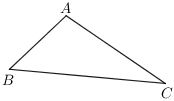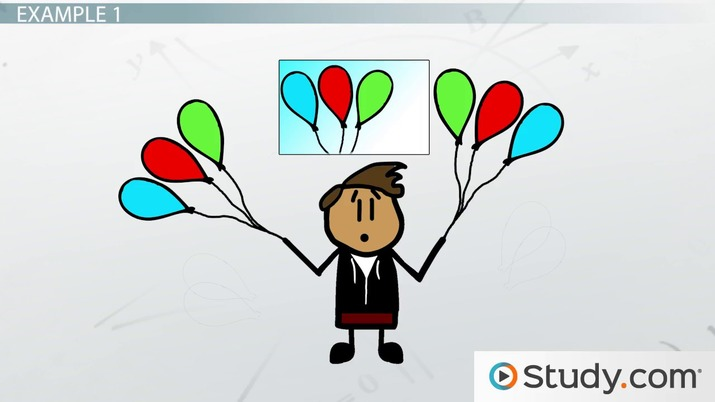# Reflexive Property of Equality

Samuel Hoyland, Yuanxin (Amy) Yang Alcocer
• Author
Samuel Hoyland

I have a bachelor's in math and music from Wesleyan University. I am currently pursuing a doctorate of music in composition at the University of Georgia. I have 9 years of work experience writing math content and 7 years of experience tutoring math one-on-one.

• Instructor
Yuanxin (Amy) Yang Alcocer

Amy has a master's degree in secondary education and has been teaching math for over 9 years. Amy has worked with students at all levels from those with special needs to those that are gifted.

Understand what the reflexive property of equality is. See how it is applied in geometry. Learn the importance of the reflexive property with the help of examples. Updated: 11/04/2021

Show

## Reflexive Property

The reflexive property of equality states that everything is equal to itself, whether it be a specific value or a mathematical expression. Examples of the reflexive property are shown below.

{eq}\hspace{2em} 3 = 3 {/eq}

{eq}\hspace{2em} -2\pi = -2\pi {/eq}

{eq}\hspace{2em} 5x^2 = 5x^2 {/eq}

{eq}\hspace{2em} 8a - b + \tfrac{1}{2}c = 8a - b + \tfrac{1}{2}c {/eq}

The name of the property comes from the word "reflection." That is because, in the reflexive property, the two sides of the equation are exact reflections of one another.### Reflexive Property in Geometry

The reflexive property is often used in proofs in geometry. In geometry, the reflexive property of congruence states that any figure (line segment, angle, polygon, 3-dimensional shape, etc.) is congruent to itself. That is, every figure is the same size and shape as itself. Though congruence does not require a figure to be in the same orientation as a figure it is congruent to, obviously, every figure also has the same orientation as itself as well.

For instance, in the diagram below, the reflexive property can be used to say that {eq}\triangle ABC \cong \triangle ABC {/eq}. Here, the symbol {eq}\cong {/eq} means "is congruent to." So, {eq}\triangle ABC \cong \triangle ABC {/eq} means that triangle {eq}ABC {/eq} is congruent to itself. In the same diagram, the reflexive property could also be used to say that each line segment is congruent to itself (e.g. {eq}\overline{AB} \cong \overline{AB} {/eq}) or each angle is congruent to itself (e.g. {eq}\angle ABC \cong \angle ABC {/eq}).There is also a reflexive property for similarity, which states that every figure is similar to itself. In geometry, similarity means that the two figures are the same shape but not necessarily the same size. So, for instance, by this property, one could write that {eq}\triangle ABC \sim \triangle ABC {/eq}, where the symbol {eq}\sim {/eq} means "is similar to."

### Importance of Reflexive Property

Although the reflexive property of equality is rarely used in algebraic manipulation, it can be used to prove other useful algebraic properties, like the addition property of equality or the multiplication property of equality. The reflexive property is more frequently used in geometry, where it is used to prove new theorems involving congruence and similarity.An error occurred trying to load this video.

Try refreshing the page, or contact customer support.

Coming up next: Addition Property of Equality: Definition & Example

### You're on a roll. Keep up the good work!

Replay
Your next lesson will play in 10 seconds
• 0:01 Definition
• 0:49 Formula
• 1:12 Example 1
• 2:01 Example 2
• 3:03 Lesson Summary
Save Save

Want to watch this again later?

Timeline
Autoplay
Autoplay
Speed Speed

## Reflexive Property Examples

### Reflexive Property of Equality Example 1

For instance, if it is given that {eq}a=b {/eq}, then the reflexive property can be used to prove that {eq}ac=bc {/eq}.

For this proof, start with the reflexive property itself, which states the following.

{eq}\hspace{2em} ac=ac {/eq}

Then since {eq}a=b {/eq}, it is possible to substitute {eq}b {/eq} for {eq}a {/eq} in the equation above.

{eq}\hspace{2em} ac=bc {/eq}

This theorem (that is, the idea that if {eq}a=b {/eq}, then {eq}ac=bc {/eq} must be true as well) is called the multiplication property of equality.

To unlock this lesson you must be a Study.com Member.

#### What are reflexive relations examples?

Some examples of reflexive relations are the equations 3 = 3, -0.21 = -0.21, 5x^2 = 5x^2, and 8a-b+1/2c = 8a-b+1/2c.

#### What is a reflexive property?

The reflexive property of equality states that everything is equal to itself, whether it be a specific value or a mathematical expression.

### Register to view this lesson

Are you a student or a teacher?